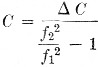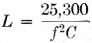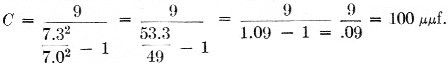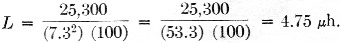# Finding tuned circuit values

Home - Techniek - Electronica - Radiotechniek - Radio amateur bladen - QST - Finding tuned circuit values

Tuned circuits in some equipment must be able to tune over specific frequency ranges. The question arises, what size coil and capacitor combination will be needed? Two simple formulas will answer this question. The first formula is:where f1 is the lowest frequency desired in Mc, f2 is the highest frequency desired in Mc, ΔC is the range of the variable capacitor in the circuit and C is the total circuit capacitance in pF at f2. The second formula is:where L is the inductance of the coil in µH, f is the highest frequency desired in Mc, and C is the capacitance found from the first formula.

The above formulas will indicate how much coil inductance and how much capacitance are necessary in order to tune the desired range with a given variable capacitor. An example of putting the formulas to work: On hand is a 1 to 10 pF variable capacitor that is to be used in a 40 meter bandspread circuit. What value of coil will be needed? Substituting in the first formula:Now to find the value of L, we substitute in the second formula:The value of C obtained by this method includes the capacitance that tubes and wiring contribute, so these should be subtracted from the calculated figure in selecting the shunt capacitor.

Sol Davis, WSWPN.